JEE  >  VITEEE Maths Test - 7

# VITEEE Maths Test - 7

Test Description

## 40 Questions MCQ Test VITEEE: Subject Wise and Full Length MOCK Tests | VITEEE Maths Test - 7

VITEEE Maths Test - 7 for JEE 2023 is part of VITEEE: Subject Wise and Full Length MOCK Tests preparation. The VITEEE Maths Test - 7 questions and answers have been prepared according to the JEE exam syllabus.The VITEEE Maths Test - 7 MCQs are made for JEE 2023 Exam. Find important definitions, questions, notes, meanings, examples, exercises, MCQs and online tests for VITEEE Maths Test - 7 below.
Solutions of VITEEE Maths Test - 7 questions in English are available as part of our VITEEE: Subject Wise and Full Length MOCK Tests for JEE & VITEEE Maths Test - 7 solutions in Hindi for VITEEE: Subject Wise and Full Length MOCK Tests course. Download more important topics, notes, lectures and mock test series for JEE Exam by signing up for free. Attempt VITEEE Maths Test - 7 | 40 questions in 50 minutes | Mock test for JEE preparation | Free important questions MCQ to study VITEEE: Subject Wise and Full Length MOCK Tests for JEE Exam | Download free PDF with solutions
 1 Crore+ students have signed up on EduRev. Have you?
VITEEE Maths Test - 7 - Question 1

### Let z₁ and z₂ be nth roots of unity which subtend a right angle at the origin, then n must be of the form :

VITEEE Maths Test - 7 - Question 2

### The least value of a for which the equation 4/sin x + 1/1 - sin x = a has atleast one solution on the interval (0, π/2) is

VITEEE Maths Test - 7 - Question 3

### The area bounded by the curve y = 4x - x2 and x-axis is

VITEEE Maths Test - 7 - Question 4

If 1 + i is the conjugate of (x+iy)(1-2i), then

VITEEE Maths Test - 7 - Question 5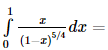VITEEE Maths Test - 7 - Question 6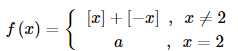where [x] denotes the greatest integer less than or equal to x, is continuous at x = 2, then the value of a is equal to

VITEEE Maths Test - 7 - Question 7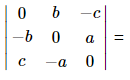=

VITEEE Maths Test - 7 - Question 8

The real part of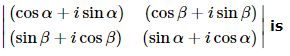is

VITEEE Maths Test - 7 - Question 9

If y' = x-y/x+y, then its solution is

VITEEE Maths Test - 7 - Question 10

The solution of the equation cosylog(secx+tanx)dx=cosxlog(secy+tany)dy is

VITEEE Maths Test - 7 - Question 11

(d2y/dx2)2 + x(dy/dx)3 is a differential equation of

VITEEE Maths Test - 7 - Question 12

If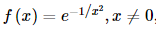and f 0 = 0 then f ′ 0 is

Detailed Solution for VITEEE Maths Test - 7 - Question 12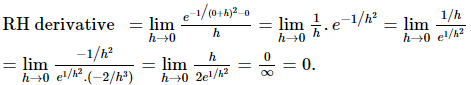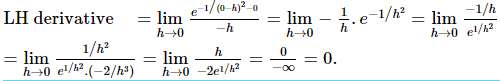VITEEE Maths Test - 7 - Question 13

Integrating factor of dy/dx+y/x=x3-3 is

VITEEE Maths Test - 7 - Question 14

For real values of x the minimum value of [(1-x+x2)/(1+x+x2)] is

VITEEE Maths Test - 7 - Question 15
If X = {1, 2, 3, 4}, then one-one onto mappings f : X → X such that f(1) = 1, f (2) ≠ 2 and f(4) ≠ 4 are given by
VITEEE Maths Test - 7 - Question 16

If ∫exsinx dx =(1/2)ex(a+c), then a=

VITEEE Maths Test - 7 - Question 17

∫ (sin -1 x + cos−1 x)   d x = ?

VITEEE Maths Test - 7 - Question 18

The solution of sin-1(2a/1 + a2) - cos-1 (1 - b2/1 + b2) = tan-1(2x/1 - x2) is :

VITEEE Maths Test - 7 - Question 19

The value of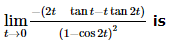VITEEE Maths Test - 7 - Question 20

If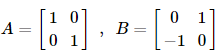and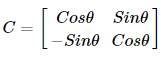, then which one of the following is true ?

VITEEE Maths Test - 7 - Question 21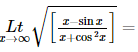VITEEE Maths Test - 7 - Question 22
Which of the following is not a statement ?
VITEEE Maths Test - 7 - Question 23

If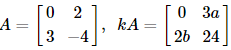, then the values of k, a, b are respectively

VITEEE Maths Test - 7 - Question 24

If 2nC3:nC2=44:3.For which value of r, nCr=15?

VITEEE Maths Test - 7 - Question 25

One hundred identical coins, each with probability p, of showing up heads are tossed. If 0 < p < 1 and the probability of heads showing on 50 coins is equal to that of the heads showing in 51 coins, then the value of p is

VITEEE Maths Test - 7 - Question 26

India plays two matches each with Australia and Pakistan. The probability of India getting points 0,1,2 are 0.45, 0.05, 0.50 . Find the probability of India getting at least 7 points in the series

VITEEE Maths Test - 7 - Question 27

A man is known to speak truth 3 out of 4 times. He throws a die and reports that it is a six. The probability that it is actually a six is

VITEEE Maths Test - 7 - Question 28

If in a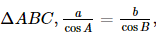, then

Detailed Solution for VITEEE Maths Test - 7 - Question 28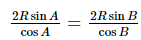or 2 sin A cos B = cos A sin B + sin A cos B = sin A + B = sin C .

VITEEE Maths Test - 7 - Question 29

The sum of the series 0.4 + 0.004 + 0.00004 + .... ∞ is

VITEEE Maths Test - 7 - Question 30

Consider the following statements:
1. Mode can be computed from histogram
2. Median is not independent of change of scale
3. Variance is independent of change of origin and scale.
Which of these is/are correct?

VITEEE Maths Test - 7 - Question 31

Covariance (x, y) between x and y if ∑x = 15, ∑y = 40, ∑xy = 110, n = 5 is

VITEEE Maths Test - 7 - Question 32

If the product of roots of the equation mx2+6x+(2m-1)=0 is -1, then the value of m is

VITEEE Maths Test - 7 - Question 33

If x2+2x+2xy+my-3 has two rational factors the value of m is

VITEEE Maths Test - 7 - Question 34

The direction cosines of the normal to the plane 6x-3y-2z=1 are

VITEEE Maths Test - 7 - Question 35

If P be the (2,6,3), then the equation of the plane thro' P at right angles to OP,O being the origin, is

VITEEE Maths Test - 7 - Question 36

The equation
3(x2 + y2) + 5x - 7y - 2 = 0 is represents

VITEEE Maths Test - 7 - Question 37

The general solution of tan3x=1 is

VITEEE Maths Test - 7 - Question 38

The radius of the circle inscribed in the triangle formed by lines x = 0, y = 0,
4x + 3y - 24 = 0 is

VITEEE Maths Test - 7 - Question 39

The median AD of the triangle ABC is bisected at E, BE meets AC in F, than AF:AC =

VITEEE Maths Test - 7 - Question 40

If p=i-2j+3k and q=3i+j+2k, then a vector r which is a linear combination of p and q and perpendicular to q is

## VITEEE: Subject Wise and Full Length MOCK Tests

1 videos|3 docs|73 tests
 Use Code STAYHOME200 and get INR 200 additional OFF Use Coupon Code
Information about VITEEE Maths Test - 7 Page
In this test you can find the Exam questions for VITEEE Maths Test - 7 solved & explained in the simplest way possible. Besides giving Questions and answers for VITEEE Maths Test - 7, EduRev gives you an ample number of Online tests for practice

## VITEEE: Subject Wise and Full Length MOCK Tests

1 videos|3 docs|73 tests